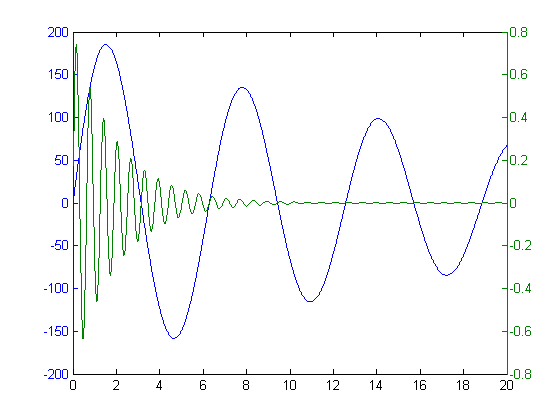## Loren on the Art of MATLABTurn ideas into MATLAB

Note

Loren on the Art of MATLAB has been archived and will not be updated.

# Multiple Y Axes

We were musing here about how common it is to want more than two Y axes on a plot. Checking out the File Exchange, there seem to be several candidates, indicating that this is something at least some people find useful.

### Contents

#### Sample Plot

Here's a sample plot using plotyy that comes with MATLAB.

x = 0:0.01:20;
y1 = 200*exp(-0.05*x).*sin(x);
y2 = 0.8*exp(-0.5*x).*sin(10*x);
plotyy(x,y1,x,y2,'plot');#### List of Some Possibilities

In addition to plotyy in MATLAB, here's a list of some of the candidates from the File Exchange.

#### What are You Plotting with More Y Axes?

I am curious to know what kind of data or results you are plotting so that having multiple y-axes makes a compelling presentation. Let us know here.

Published with MATLAB® R2013a

### コメント

コメントを残すには、ここ をクリックして MathWorks アカウントにサインインするか新しい MathWorks アカウントを作成します。